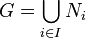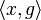# 2-Engel group

## Definition

No. Shorthand A group is termed a Levi group or a 2-Engel group if ... A group$G$ is termed a Levi group or 2-Engel group if ...
1 conjugates commute any two conjugate elements of the group commute.$x$ commutes with$gxg^{-1}$ for all$x,g \in G$
2 normal closures abelian the normal closure of any cyclic subgroup (or the normal subgroup generated by any one-element subset) is abelian the normal subgroup generated by$x$ is abelian for all$x \in G$.
3 union of abelian normal subgroups the group is a union (as a set) of abelian normal subgroups there is a collection of abelian normal subgroups$N_i, i \in I$ of$G$ such that$G = \bigcup_{i \in I} N_i$
4 2-local class two the 2-local nilpotency class of the group is at most 2. for any$x,g \in G$, the subgroup$\langle x,g \rangle$ is a group of class at most two.
5 2-Engel the group is a$2$-Engel group: the commutator between any element and its commutator with another element is the identity element. the commutator$[x,[x,g]]$ is the identity element for all$x,g \in G$.
6 cyclic property of triple commutators triple commutators are preserved under cyclic permutation of the inputs. for all$x,y,z \in G$, we have$[x,[y,z]] = [y,[z,x]] = [z,[x,y]]$.

## Contents

This article defines a term that has been used or referenced in a journal article or standard publication, but may not be generally accepted by the mathematical community as a standard term.[SHOW MORE]
This article defines a group property: a property that can be evaluated to true/false for any given group, invariant under isomorphism
View a complete list of group properties
VIEW RELATED: Group property implications | Group property non-implications |Group metaproperty satisfactions | Group metaproperty dissatisfactions | Group property satisfactions | Group property dissatisfactions

## Formalisms

### In terms of the Levi operator

This property is obtained by applying the Levi operator to the property: Abelian group
View other properties obtained by applying the Levi operator

## Relation with other properties

### Stronger properties

Property Meaning Proof of implication Proof of strictness (reverse implication failure) Intermediate notions
bounded Engel group$k$-Engel group for some finite$k$ |FULL LIST, MORE INFO
Engel group For any two elements$x$ and$y$, the iterated commutator of$x$ with$y$ eventually becomes trivial |FULL LIST, MORE INFO
group in which order of commutator divides order of element For any two elements$x$ and$y$, if the order of$x$ is finite, the order of$[x,y]$ divides the order of$x$ |FULL LIST, MORE INFO